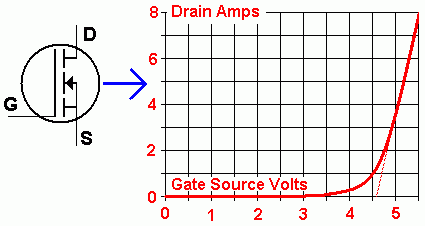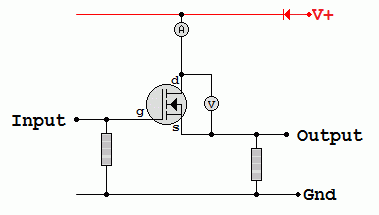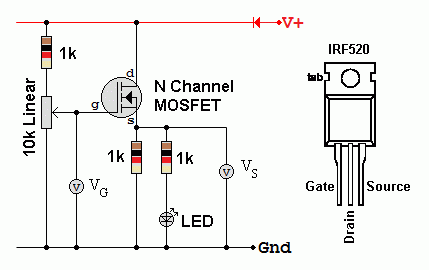RANDOM PAGE

SITE SEARCH

LOG
IN

HELP

# Source Follower

This is the AQA version closing after June 2019. Visit the the version for Eduqas instead.

## Source Follower Properties• The voltage gain is One.
• Current gain is large.
• Power gain is large.
• s = SOURCE.
• g = GATE.
• d = DRAIN.
• Extremely high input or gate resistance (Close to infinity).
• The N Channel device amplifies positive signals.
• The P Channel device amplifies negative signals.
• Ideal device: Vout = Vin.
• A 2 to 4 volt drop depending on the MOSFET.
• Real life device: Typical N Channel: Vout = Vin - 2.
• Real life device: Typical P Channel: Vout = Vin + 2.

## MOSFET CharacteristicsThis graph is for a power MOSFET such as the IRF520 device. You can see that the device does not start conducting until the gate voltage is significantly greater than 3 Volts. An ideal MOSFET would give a straight line graph starting from zero. This graph also explains the 2 to 4 Volt drop mentioned above.

## Source Follower Power CalculationsDC Power is calculated using Volts x Amps. This is the current through the MOSFET and the voltage across the MOSFET.

There are three commonly occurring states.

• The MOSFET is turned off. The current is zero and the power dissipated = zero.
• The MOSFET is saturated.
• An ideal MOSFET would have zero volts across it.
• Under these conditions the power dissipated is zero again.
• A real MOSFET might have 20 mV across it. (This will be in the data sheet or specified in the exam question).
• In this case, do the Volts x Amps calculation and expect a small answer.
• The third state is where the MOSFET is conducting and not saturated.
• This will result in the highest heat dissipation.
• Work out Volts x Amps.
• A heatsink will often be needed in higher power circuits.

## AC Power Calculations

• Use the RMS values for the voltage across the MOSFET and the current through the it.
• RMS Value = 0.7 x Peak Value

## Source Follower Experiment• Build this circuit and measure VG and VS.
• Plot a graph showing how these are related.
• Run the circuit on 12 Volts.

reviseOmatic V3     Contacts, ©, Cookies, Data Protection and Disclaimers Hosted at linode.com, London